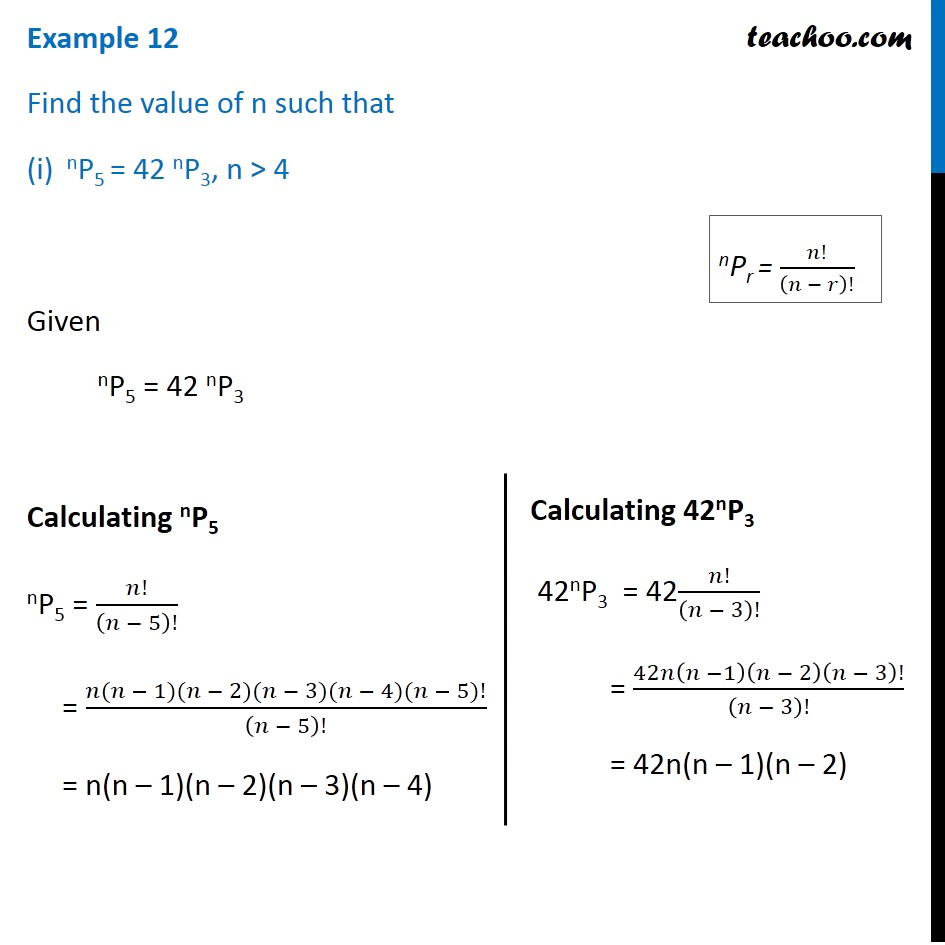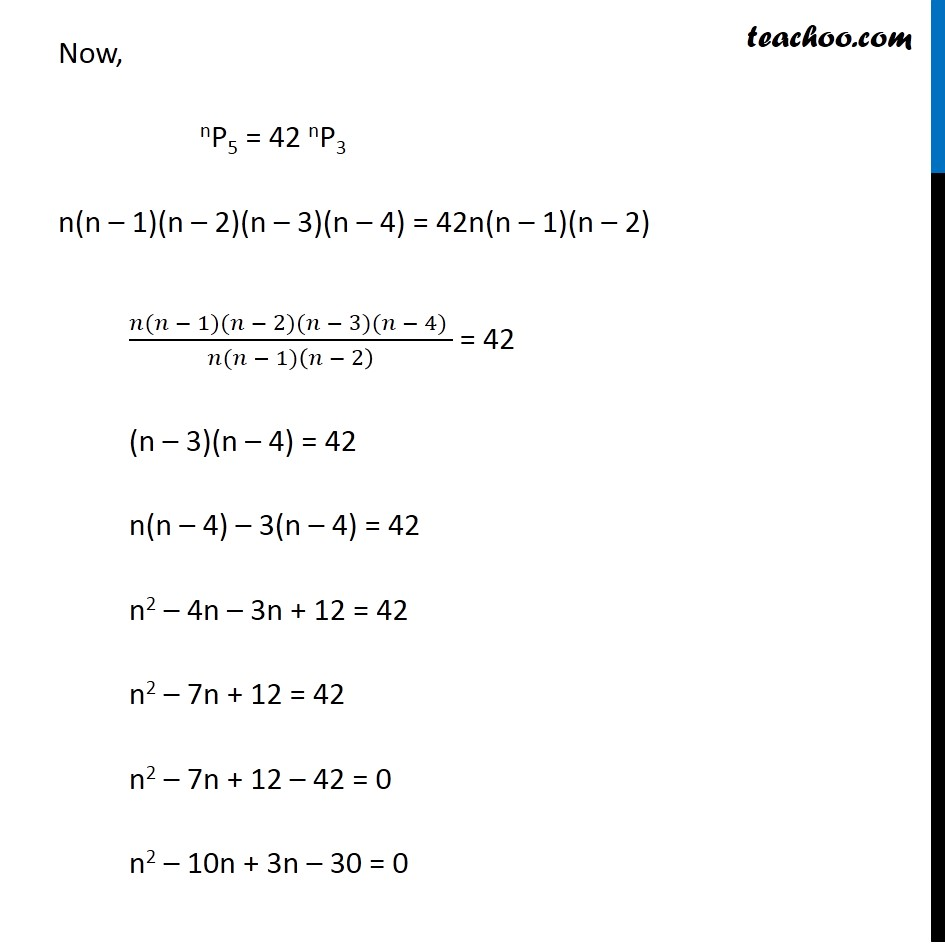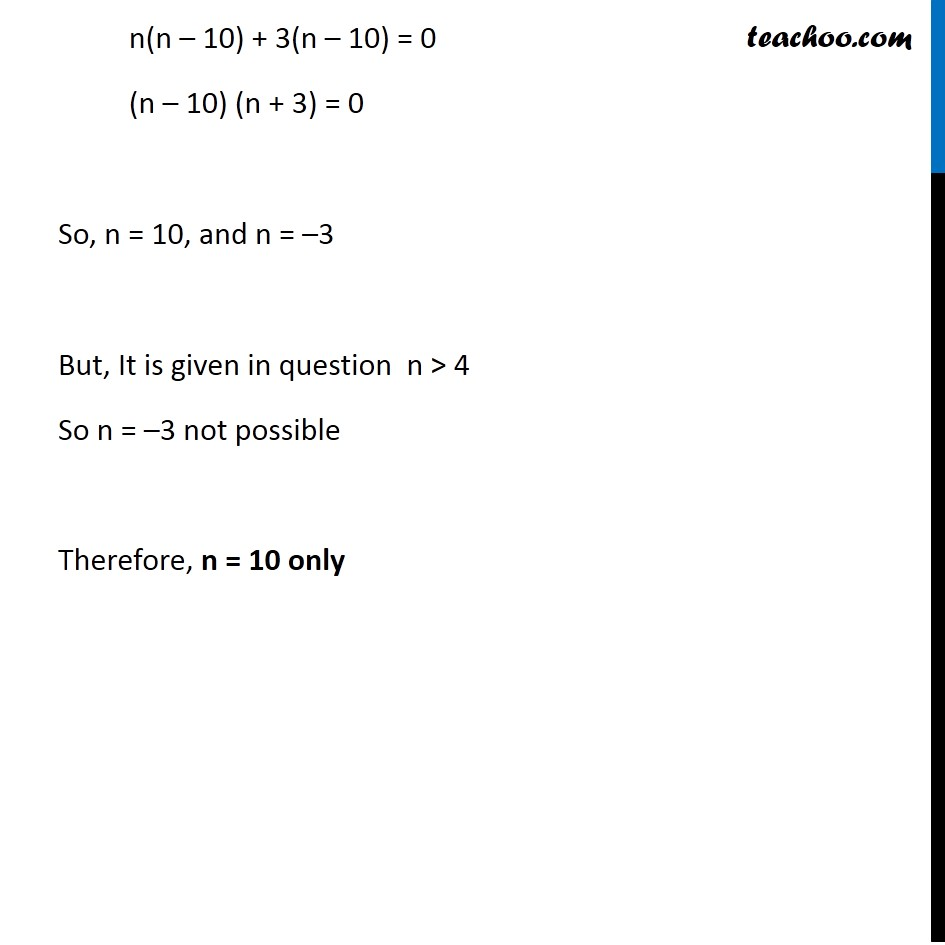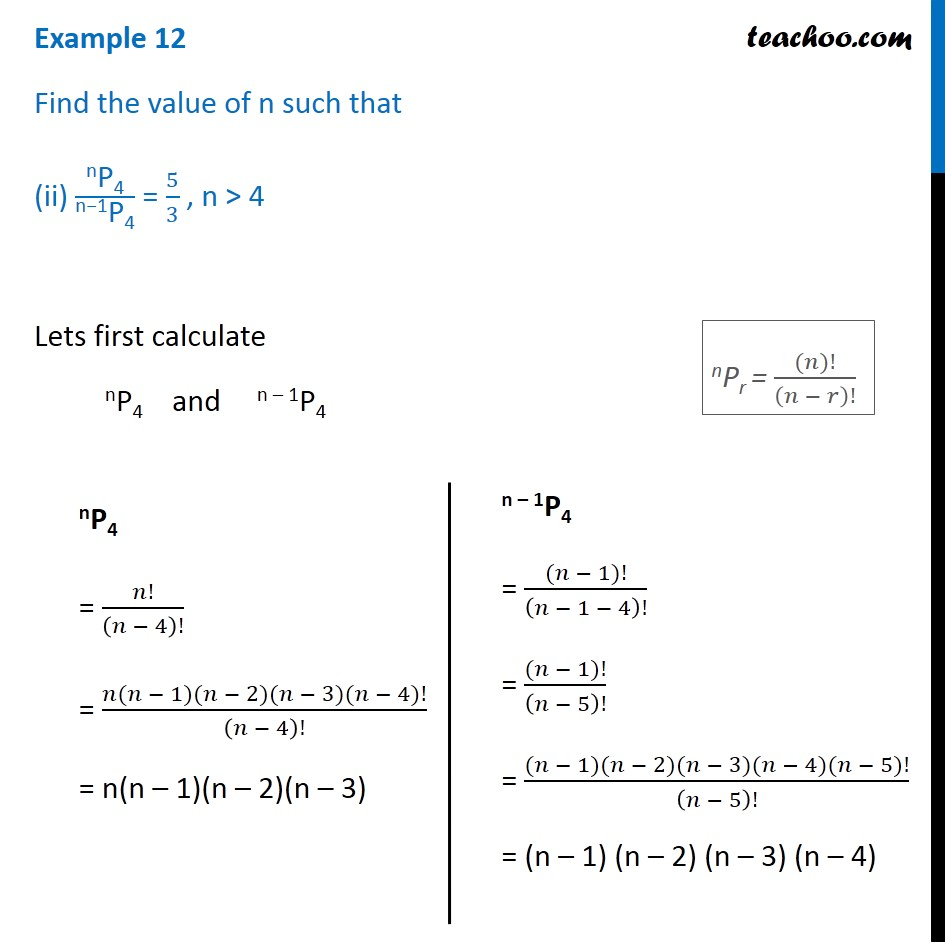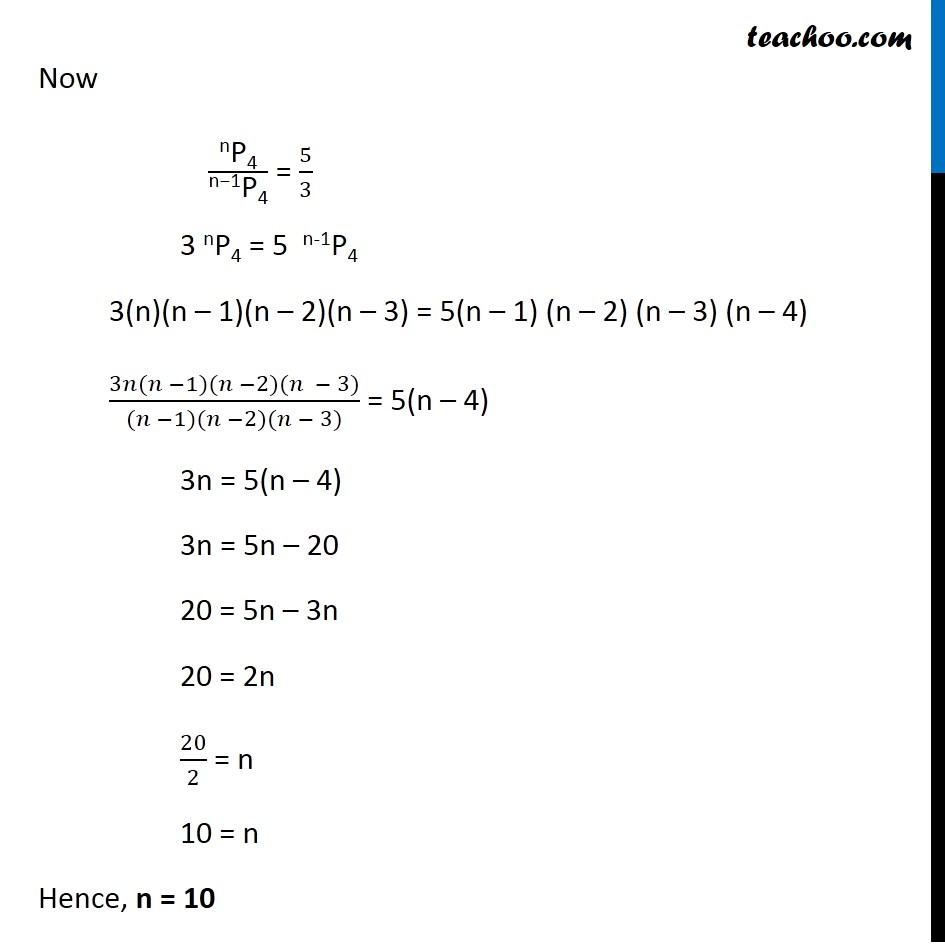Subscribe to our Youtube Channel - https://you.tube/teachoo

1. Chapter 7 Class 11 Permutations and Combinations
2. Serial order wise
3. Examples

Transcript

Example 12 Find the value of n such that nP5 = 42 nP3, n > 4 Given nP5 = 42 nP3 Calculating nP5 nP5 = 𝑛!/(𝑛 − 5)! = (𝑛(𝑛 − 1)(𝑛 − 2)(𝑛 − 3)(𝑛 − 4)(𝑛 − 5)!)/(𝑛 − 5)! = n(n – 1)(n – 2)(n – 3)(n – 4) Calculating 42nP3 42nP3 = 42𝑛!/(𝑛 − 3)! = 42𝑛(𝑛 −1)(𝑛 − 2)(𝑛 − 3)!/(𝑛 − 3)! = 42n(n – 1)(n – 2) Now, nP5 = 42 nP3 n(n – 1)(n – 2)(n – 3)(n – 4) = 42n(n – 1)(n – 2) (𝑛(𝑛 − 1)(𝑛 − 2)(𝑛 − 3)(𝑛 − 4) )/(𝑛(𝑛 − 1)(𝑛 − 2) ) = 42 (n – 3)(n – 4) = 42 n(n – 4) – 3(n – 4) = 42 n2 – 4n – 3n + 12 = 42 n2 – 7n + 12 = 42 n2 – 7n + 12 – 42 = 0 n2 – 10n + 3n – 30 = 0 n(n – 10) + 3(n – 10) = 0 (n – 10) (n + 3) = 0 So, n = 10, and n = – 3 n(n – 10) + 3(n – 10) = 0 (n – 10) (n + 3) = 0 So, n = 10, and n = –3 But, It is given in question n > 4 So n = –3 not possible Therefore, n = 10 only Example 12 Find the value of n such that (ii) "nP4" /"n−1P4" = 5/3 , n > 4 Lets first calculate nP4 and n – 1P4 nP4 = 𝑛!/(𝑛 − 4)! = (𝑛(𝑛 − 1)(𝑛 − 2)(𝑛 − 3)(𝑛 − 4)!)/(𝑛 − 4)! = n(n – 1)(n – 2)(n – 3) n – 1P4 = ((𝑛 − 1)!)/(𝑛 − 1 − 4)! = ((𝑛 − 1)!)/(𝑛 − 5)! = ((𝑛 − 1)(𝑛 − 2)(𝑛 − 3)(𝑛 − 4)(𝑛 − 5)!)/(𝑛 − 5)! = (n – 1) (n – 2) (n – 3) (n – 4) nPr = ((𝑛)!)/(𝑛 − 𝑟)! Now "nP4" /"n−1P4" = 5/3 3 nP4 = 5 n-1P4 3(n)(n – 1)(n – 2)(n – 3) = 5(n – 1) (n – 2) (n – 3) (n – 4) (3𝑛(𝑛 −1)(𝑛 −2)(𝑛 − 3))/((𝑛 −1)(𝑛 −2)(𝑛 − 3)) = 5(n – 4) 3n = 5(n – 4) 3n = 5n – 20 20 = 5n – 3n 20 = 2n 20/2 = n 10 = n Hence, n = 10 n(n – 10) + 3(n – 10) = 0 (n – 10) (n + 3) = 0 So, n = 10, and n = –3 But, It is given in question n > 4 So n = –3 not possible Therefore, n = 10 only Example 12 Find the value of n such that (ii) "nP4" /"n−1P4" = 5/3 , n > 4 Lets first calculate nP4 and n – 1P4 nP4 = 𝑛!/(𝑛 − 4)! = (𝑛(𝑛 − 1)(𝑛 − 2)(𝑛 − 3)(𝑛 − 4)!)/(𝑛 − 4)! = n(n – 1)(n – 2)(n – 3) n – 1P4 = ((𝑛 − 1)!)/(𝑛 − 1 − 4)! = ((𝑛 − 1)!)/(𝑛 − 5)! = ((𝑛 − 1)(𝑛 − 2)(𝑛 − 3)(𝑛 − 4)(𝑛 − 5)!)/(𝑛 − 5)! = (n – 1) (n – 2) (n – 3) (n – 4) nPr = ((𝑛)!)/(𝑛 − 𝑟)! Now "nP4" /"n−1P4" = 5/3 3 nP4 = 5 n-1P4 3(n)(n – 1)(n – 2)(n – 3) = 5(n – 1) (n – 2) (n – 3) (n – 4) (3𝑛(𝑛 −1)(𝑛 −2)(𝑛 − 3))/((𝑛 −1)(𝑛 −2)(𝑛 − 3)) = 5(n – 4) 3n = 5(n – 4) 3n = 5n – 20 20 = 5n – 3n 20 = 2n 20/2 = n 10 = n Hence, n = 10

Examples